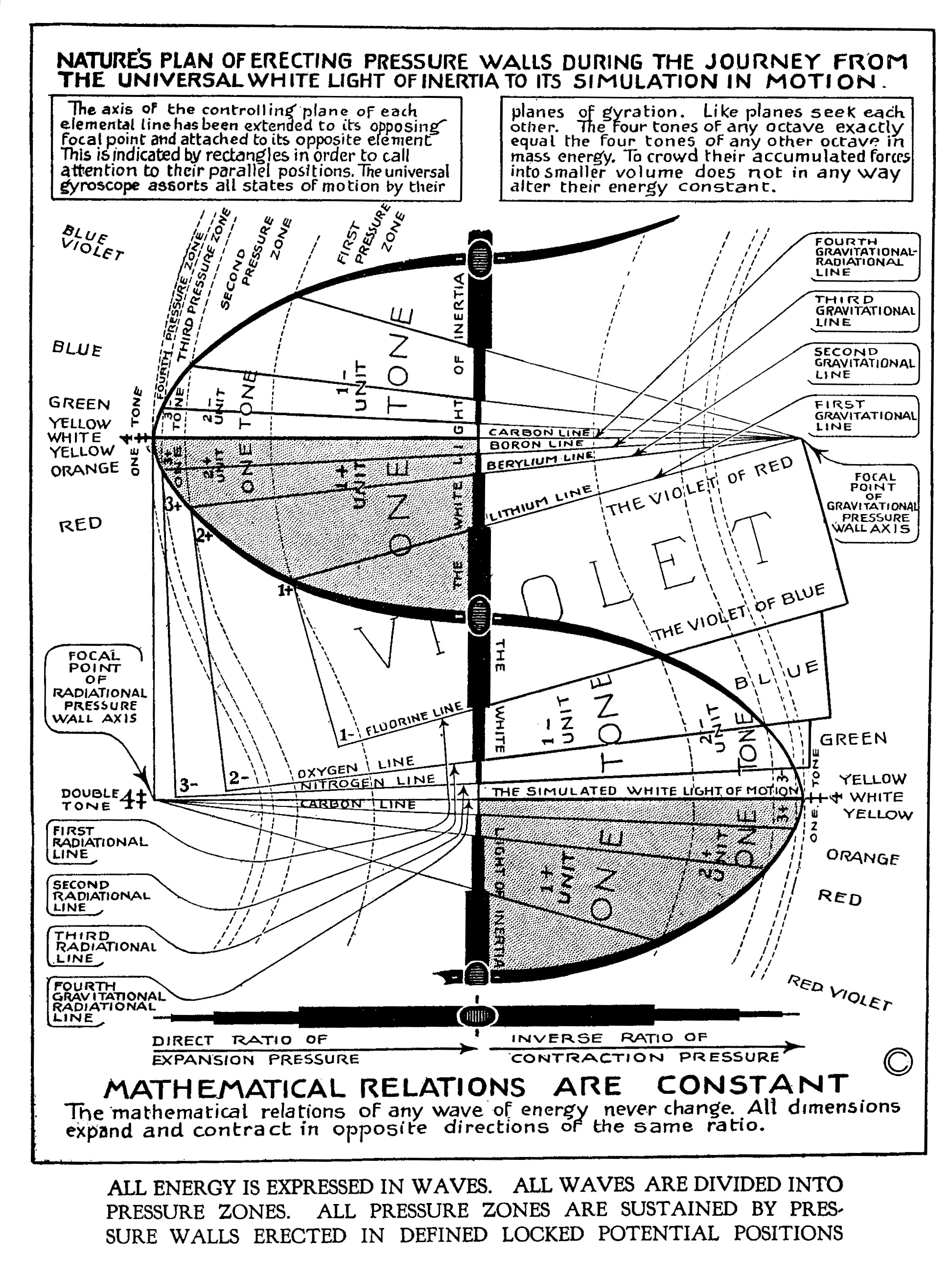# Mathematical Relations are Constant - page 125"NATURE'S PLAN OF ERECTING PRESSURE WALLS DURING THE JOURNEY FROM THE UNIVERSAL WHITE LIGHT OF INERTIA TO ITS SIMULATION IN MOTION" [Mathematical Relations are Constant]

"The axis of the controlling plane of each elemental line has been extended to its opposing focal point and attached to its opposite element. This is indicated by rectangles in order to call attention to their parallel positions. The universal gyroscope assorts all states of motion by their planes of gyration. Like planes seek each other. The four tones of any octave exactly equal four tones of any other octave in mass energy. To crowd their accumulated forces into smaller volume does not in any way alter their energy." [Mathematical Relations are Constant]

"Mathematical Relations are Constant
The mathematical relations of any wave of energy never change. All dimensions expand and contract in opposite directions of the same ratio." [Mathematical Relations are Constant]

"ALL ENERGY IS EXPRESSED IN WAVES. ALL WAVES ARE DIVIDED INTO PRESSURE ZONES. ALL PRESSURE ZONES ARE SUSTAINED BY PRESSURE WALLS ERECTED IN DEFINED LOCKED POTENTIAL POSITIONS" [Mathematical Relations are Constant]

Professor Daniel Brinton
"The rhythmic relations in which force acts are everywhere, under all conditions, and at all times, the same. They are found experimentally to be universally expressible by the mathematical relations of thirds.

"These threefold relations may be expressed with regard to their results as,

"From these three actions are derived the three fundamental LAWS OF BEING:

I. Law of Assimilation: every individualized object assimilates itself to all other objects.

II. Law of Individualization: every such object tends to assimilate all other objects to itself.

III. Law of the Dominant: every such object is such by virtue of the higher or dominant force which controls these (above) two tendencies. [14.09 - Brintons Laws of Being]